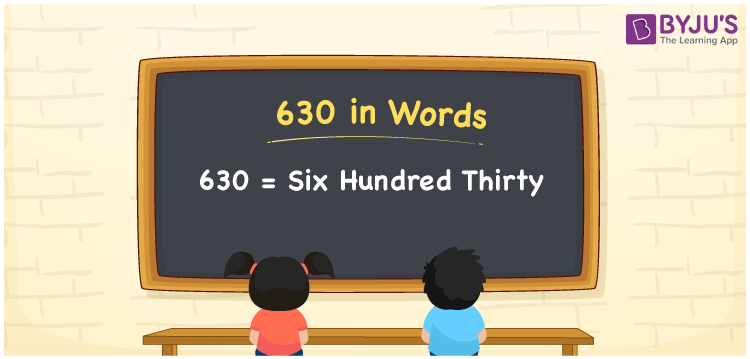# 630 in Words

630 in words can be written as Six Hundred Thirty. You will learn here about why writing the numbers in words is important in our daily activities. For instance, if you want to draw Rs. 630 from the bank, then you can say that “I want to draw Six Hundred Thirty Rupees from the bank”. For more details of the numbers in words topic, students can refer to this article. Hence, 630 can be read as “Six Hundred Thirty” in English.

 630 in words Six Hundred Thirty Six Hundred Thirty in Numbers 630

## 630 in English Words## How to Write 630 in Words?

The place value chart of 630 is provided below for the reference of the students. The number 630 has three digits and the place value for each digit is represented in the table here.

 Hundreds Tens Ones 6 3 0

The expanded form of 630 is:

6 × Hundred + 3 × Ten + 0 × One

= 6 × 100 + 3 × 10 + 0 × 1

= 600 + 30

= 630

= Six Hundred Thirty

Therefore, 630 in words is written as Six Hundred Thirty.

630 is a natural number that precedes 631 and succeeds 629.

630 in words – Six Hundred Thirty

Is 630 an odd number? – No

Is 630 an even number? – Yes

Is 630 a perfect square number? – No

Is 630 a perfect cube number? – No

Is 630 a prime number? – No

Is 630 a composite number? – Yes

## Frequently Asked Questions on 630 in Words

Q1

### Write 630 in words.

630 can be written in words as “Six Hundred Thirty”.
Q2

### How do you write Six Hundred Thirty in numbers?

Six Hundred Thirty can be written in numbers as 630.
Q3

### Is 630 a perfect cube number?

No, 630 is not a perfect cube number as it is not the product of three similar numbers.